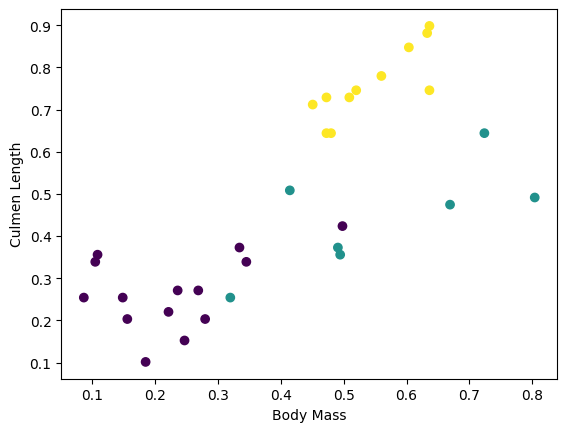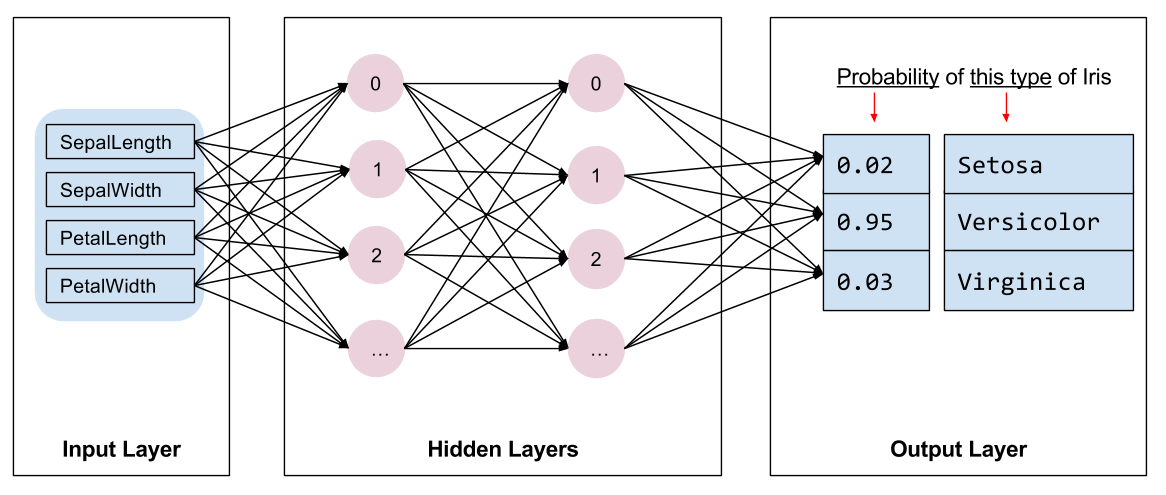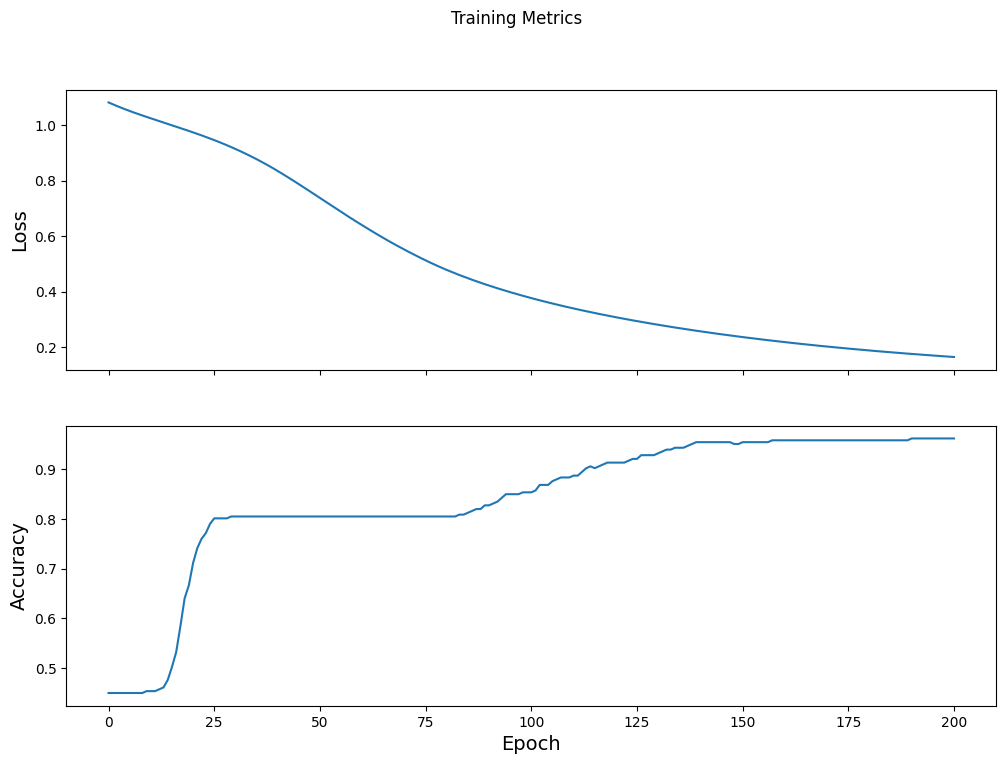# 自定义训练: 演示

1. 构建一个模型，
2. 用样例数据集对模型进行训练，以及
3. 利用该模型对未知数据进行预测。

## TensorFlow 编程

1. 数据集的导入与解析
2. 选择模型类型
3. 对模型进行训练
4. 评估模型效果
5. 使用训练过的模型进行预测

## 环境的搭建

### 配置导入

``````import os
import matplotlib.pyplot as plt
``````
``````import tensorflow as tf
``````
``````print("TensorFlow version: {}".format(tf.__version__))
print("Eager execution: {}".format(tf.executing_eagerly()))
``````
```TensorFlow version: 2.9.1
Eager execution: True
```

## 鸢尾花分类问题

• 山鸢尾
• 维吉尼亚鸢尾
• 变色鸢尾Figure 1. 山鸢尾 (by Radomil, CC BY-SA 3.0), 变色鸢尾, (by Dlanglois, CC BY-SA 3.0), and 维吉尼亚鸢尾 (by Frank Mayfield, CC BY-SA 2.0).{nbsp}

## 导入和解析训练数据集

### 下载数据集

``````train_dataset_url = "https://storage.googleapis.com/download.tensorflow.org/data/iris_training.csv"

train_dataset_fp = tf.keras.utils.get_file(fname=os.path.basename(train_dataset_url),
origin=train_dataset_url)

print("Local copy of the dataset file: {}".format(train_dataset_fp))
``````
```Local copy of the dataset file: /home/kbuilder/.keras/datasets/iris_training.csv
```

### 检查数据

````head -n5 {train_dataset_fp}`
```
```120,4,setosa,versicolor,virginica
6.4,2.8,5.6,2.2,2
5.0,2.3,3.3,1.0,1
4.9,2.5,4.5,1.7,2
4.9,3.1,1.5,0.1,0
```

1. 第一行是表头，其中包含数据集信息：
• 共有 120 个样本。每个样本都有四个特征和一个标签名称，标签名称有三种可能。
1. 后面的行是数据记录，每个样本各占一行，其中：
• 前四个字段是特征: 这四个字段代表的是样本的特点。在此数据集中，这些字段存储的是代表花卉测量值的浮点数。
• 最后一列是标签:即我们想要预测的值。对于此数据集，该值为 0、1 或 2 中的某个整数值（每个值分别对应一个花卉名称）。

``````# column order in CSV file
column_names = ['sepal_length', 'sepal_width', 'petal_length', 'petal_width', 'species']

feature_names = column_names[:-1]
label_name = column_names[-1]

print("Features: {}".format(feature_names))
print("Label: {}".format(label_name))
``````
```Features: ['sepal_length', 'sepal_width', 'petal_length', 'petal_width']
Label: species
```

• `0` : 山鸢尾
• `1` : 变色鸢尾
• `2` : 维吉尼亚鸢尾

``````class_names = ['Iris setosa', 'Iris versicolor', 'Iris virginica']
``````

### 创建一个 `tf.data.Dataset`

TensorFlow的 Dataset API 可处理在向模型加载数据时遇到的许多常见情况。这是一种高阶 API ，用于读取数据并将其转换为可供训练使用的格式。如需了解详情，请参阅数据集快速入门指南

``````batch_size = 32

train_dataset = tf.data.experimental.make_csv_dataset(
train_dataset_fp,
batch_size,
column_names=column_names,
label_name=label_name,
num_epochs=1)
``````

`make_csv_dataset` 返回一个`(features, label)` 对构建的 `tf.data.Dataset` ，其中 `features` 是一个字典: `{'feature_name': value}`

``````features, labels = next(iter(train_dataset))

print(features)
``````
```OrderedDict([('sepal_length', <tf.Tensor: shape=(32,), dtype=float32, numpy=
array([4.8, 4.6, 5. , 6.5, 5. , 5.6, 6.9, 7. , 5. , 4.9, 5.8, 7.7, 6.3,
6.3, 6. , 6.8, 5.4, 6.7, 4.9, 7.2, 5.8, 4.8, 6.9, 5.8, 5. , 6.4,
6.1, 6.1, 6.1, 6.7, 6. , 5.4], dtype=float32)>), ('sepal_width', <tf.Tensor: shape=(32,), dtype=float32, numpy=
array([3.1, 3.1, 3.5, 3. , 3.5, 2.5, 3.2, 3.2, 3.4, 3.1, 2.7, 2.6, 2.7,
3.3, 3. , 3.2, 3.7, 3.1, 3. , 3. , 2.6, 3. , 3.1, 2.7, 2. , 3.1,
2.9, 2.6, 2.8, 3. , 2.9, 3.9], dtype=float32)>), ('petal_length', <tf.Tensor: shape=(32,), dtype=float32, numpy=
array([1.6, 1.5, 1.3, 5.8, 1.6, 3.9, 5.7, 4.7, 1.6, 1.5, 4.1, 6.9, 4.9,

6. , 4.8, 5.9, 1.5, 5.6, 1.4, 5.8, 4. , 1.4, 4.9, 5.1, 3.5, 5.5,
4.7, 5.6, 4. , 5. , 4.5, 1.7], dtype=float32)>), ('petal_width', <tf.Tensor: shape=(32,), dtype=float32, numpy=
array([0.2, 0.2, 0.3, 2.2, 0.6, 1.1, 2.3, 1.4, 0.4, 0.1, 1. , 2.3, 1.8,
2.5, 1.8, 2.3, 0.2, 2.4, 0.2, 1.6, 1.2, 0.1, 1.5, 1.9, 1. , 1.8,
1.4, 1.4, 1.3, 1.7, 1.5, 0.4], dtype=float32)>)])
```

``````plt.scatter(features['petal_length'],
features['sepal_length'],
c=labels,
cmap='viridis')

plt.xlabel("Petal length")
plt.ylabel("Sepal length")
plt.show()
````````````def pack_features_vector(features, labels):
"""Pack the features into a single array."""
features = tf.stack(list(features.values()), axis=1)
return features, labels
``````

``````train_dataset = train_dataset.map(pack_features_vector)
``````

`Dataset` 的特征元素被构成了形如 `(batch_size, num_features)` 的数组。我们来看看前几个样本:

``````features, labels = next(iter(train_dataset))

print(features[:5])
``````
```tf.Tensor(
[[7.  3.2 4.7 1.4]
[7.7 2.8 6.7 2. ]
[5.9 3.  5.1 1.8]
[6.4 2.7 5.3 1.9]
[5.7 4.4 1.5 0.4]], shape=(5, 4), dtype=float32)
```

## 选择模型类型

### 选择模型图 2. 包含特征、隐藏层和预测的神经网络{nbsp}

### 使用 Keras 创建模型

TensorFlow tf.keras API 是创建模型和层的首选方式。通过该 API，您可以轻松地构建模型并进行实验，而将所有部分连接在一起的复杂工作则由 Keras 处理。

tf.keras.Sequential 模型是层的线性堆叠。该模型的构造函数会采用一系列层实例；在本示例中，采用的是 2 个密集层（各自包含10个节点）,以及 1 个输出层（包含 3 个代表标签预测的节点。第一个层的 `input_shape` 参数对应该数据集中的特征数量，它是一项必需参数：

``````model = tf.keras.Sequential([
tf.keras.layers.Dense(10, activation=tf.nn.relu, input_shape=(4,)),  # input shape required
tf.keras.layers.Dense(10, activation=tf.nn.relu),
tf.keras.layers.Dense(3)
])
``````

### 使用模型

``````predictions = model(features)
predictions[:5]
``````
```<tf.Tensor: shape=(5, 3), dtype=float32, numpy=
array([[ 1.7844266 , -2.2687607 ,  0.2002536 ],
[ 2.0090103 , -2.8222938 ,  0.40188438],
[ 1.740761  , -2.3017416 ,  0.32230324],
[ 1.7462329 , -2.3763986 ,  0.31881684],
[ 1.9809153 , -2.3277717 , -0.8571239 ]], dtype=float32)>
```

``````tf.nn.softmax(predictions[:5])
``````
```<tf.Tensor: shape=(5, 3), dtype=float32, numpy=
array([[0.81800646, 0.01420626, 0.16778733],
[0.8275139 , 0.00660034, 0.16588585],
[0.79387724, 0.01393532, 0.19218744],
[0.79609597, 0.0128982 , 0.19100578],
[0.93284315, 0.01254786, 0.05460896]], dtype=float32)>
```

``````print("Prediction: {}".format(tf.argmax(predictions, axis=1)))
print("    Labels: {}".format(labels))
``````
```Prediction: [0 0 0 0 0 0 0 0 0 0 0 0 0 0 0 0 0 0 0 0 0 0 0 0 0 0 0 0 0 0 0 0]
Labels: [1 2 2 2 0 2 0 1 1 0 1 0 2 2 2 0 0 1 2 0 0 1 0 0 2 0 0 2 1 2 0 1]
```

## 训练模型

### 定义损失和梯度函数

``````loss_object = tf.keras.losses.SparseCategoricalCrossentropy(from_logits=True)
``````
``````def loss(model, x, y, training):
# training=training is needed only if there are layers with different
# behavior during training versus inference (e.g. Dropout).
y_ = model(x, training=training)

return loss_object(y_true=y, y_pred=y_)

l = loss(model, features, labels, training=False)
print("Loss test: {}".format(l))
``````
```Loss test: 1.6010572910308838
```

``````def grad(model, inputs, targets):
loss_value = loss(model, inputs, targets, training=True)
``````

### 创建优化器图 3. 优化算法在三维空间中随时间推移而变化的可视化效果。(来源: 斯坦福大学 CS231n 课程，MIT 许可证，Image credit: Alec Radford)

TensorFlow有许多可用于训练的优化算法。此模型使用的是 tf.train.GradientDescentOptimizer ， 它可以实现随机梯度下降法（SGD）。`learning_rate` 被用于设置每次迭代（向下行走）的步长。 这是一个 超参数 ，您通常需要调整此参数以获得更好的结果。

``````optimizer = tf.keras.optimizers.SGD(learning_rate=0.01)
``````

``````loss_value, grads = grad(model, features, labels)

print("Step: {}, Initial Loss: {}".format(optimizer.iterations.numpy(),
loss_value.numpy()))

print("Step: {},         Loss: {}".format(optimizer.iterations.numpy(),
loss(model, features, labels, training=True).numpy()))
``````
```Step: 0, Initial Loss: 1.6010572910308838
Step: 1,         Loss: 1.4704034328460693
```

### 训练循环

1. 迭代每个周期。通过一次数据集即为一个周期。
2. 在一个周期中，遍历训练 `Dataset` 中的每个样本，并获取样本的特征`x`）和标签`y`）。
3. 根据样本的特征进行预测，并比较预测结果和标签。衡量预测结果的不准确性，并使用所得的值计算模型的损失和梯度。
4. 使用 `optimizer` 更新模型的变量。
5. 跟踪一些统计信息以进行可视化。
6. 对每个周期重复执行以上步骤。

`num_epochs` 变量是遍历数据集集合的次数。与直觉恰恰相反的是，训练模型的时间越长，并不能保证模型就越好。`num_epochs` 是一个可以调整的超参数。选择正确的次数通常需要一定的经验和实验基础。

``````## Note: Rerunning this cell uses the same model variables

# Keep results for plotting
train_loss_results = []
train_accuracy_results = []

num_epochs = 201

for epoch in range(num_epochs):
epoch_loss_avg = tf.keras.metrics.Mean()
epoch_accuracy = tf.keras.metrics.SparseCategoricalAccuracy()

# Training loop - using batches of 32
for x, y in train_dataset:
# Optimize the model

# Track progress
epoch_loss_avg.update_state(loss_value)  # Add current batch loss
# Compare predicted label to actual label
# training=True is needed only if there are layers with different
# behavior during training versus inference (e.g. Dropout).
epoch_accuracy.update_state(y, model(x, training=True))

# End epoch
train_loss_results.append(epoch_loss_avg.result())
train_accuracy_results.append(epoch_accuracy.result())

if epoch % 50 == 0:
print("Epoch {:03d}: Loss: {:.3f}, Accuracy: {:.3%}".format(epoch,
epoch_loss_avg.result(),
epoch_accuracy.result()))
``````
```Epoch 000: Loss: 1.495, Accuracy: 35.000%
Epoch 050: Loss: 0.533, Accuracy: 80.833%
Epoch 100: Loss: 0.342, Accuracy: 90.833%
Epoch 150: Loss: 0.206, Accuracy: 97.500%
Epoch 200: Loss: 0.145, Accuracy: 98.333%
```

### 可视化损失函数随时间推移而变化的情况

``````fig, axes = plt.subplots(2, sharex=True, figsize=(12, 8))
fig.suptitle('Training Metrics')

axes.set_ylabel("Loss", fontsize=14)
axes.plot(train_loss_results)

axes.set_ylabel("Accuracy", fontsize=14)
axes.set_xlabel("Epoch", fontsize=14)
axes.plot(train_accuracy_results)
plt.show()
``````## 评估模型的效果

5.9 3.0 4.3 1.5 1 1
6.9 3.1 5.4 2.1 2 2
5.1 3.3 1.7 0.5 0 0
6.0 3.4 4.5 1.6 1 2
5.5 2.5 4.0 1.3 1 1

{nbsp}

### 建立测试数据集

``````test_url = "https://storage.googleapis.com/download.tensorflow.org/data/iris_test.csv"

test_fp = tf.keras.utils.get_file(fname=os.path.basename(test_url),
origin=test_url)
``````
``````test_dataset = tf.data.experimental.make_csv_dataset(
test_fp,
batch_size,
column_names=column_names,
label_name='species',
num_epochs=1,
shuffle=False)

test_dataset = test_dataset.map(pack_features_vector)
``````

### 根据测试数据集评估模型

``````test_accuracy = tf.keras.metrics.Accuracy()

for (x, y) in test_dataset:
# training=False is needed only if there are layers with different
# behavior during training versus inference (e.g. Dropout).
logits = model(x, training=False)
prediction = tf.argmax(logits, axis=1, output_type=tf.int32)
test_accuracy(prediction, y)

print("Test set accuracy: {:.3%}".format(test_accuracy.result()))
``````
```Test set accuracy: 96.667%
```

``````tf.stack([y,prediction],axis=1)
``````
```<tf.Tensor: shape=(30, 2), dtype=int32, numpy=
array([[1, 1],
[2, 2],
[0, 0],
[1, 1],
[1, 1],
[1, 1],
[0, 0],
[2, 2],
[1, 1],
[2, 2],
[2, 2],
[0, 0],
[2, 2],
[1, 1],
[1, 1],
[0, 0],
[1, 1],
[0, 0],
[0, 0],
[2, 2],
[0, 0],
[1, 1],
[2, 2],
[1, 2],
[1, 1],
[1, 1],
[0, 0],
[1, 1],
[2, 2],
[1, 1]], dtype=int32)>
```

## 使用经过训练的模型进行预测

• `0`: 山鸢尾
• `1`: 变色鸢尾
• `2`: 维吉尼亚鸢尾
``````predict_dataset = tf.convert_to_tensor([
[5.1, 3.3, 1.7, 0.5,],
[5.9, 3.0, 4.2, 1.5,],
[6.9, 3.1, 5.4, 2.1]
])

# training=False is needed only if there are layers with different
# behavior during training versus inference (e.g. Dropout).
predictions = model(predict_dataset, training=False)

for i, logits in enumerate(predictions):
class_idx = tf.argmax(logits).numpy()
p = tf.nn.softmax(logits)[class_idx]
name = class_names[class_idx]
print("Example {} prediction: {} ({:4.1f}%)".format(i, name, 100*p))
``````
```Example 0 prediction: Iris setosa (97.6%)
Example 1 prediction: Iris versicolor (81.6%)
Example 2 prediction: Iris virginica (78.6%)
```
[{ "type": "thumb-down", "id": "missingTheInformationINeed", "label":"没有我需要的信息" },{ "type": "thumb-down", "id": "tooComplicatedTooManySteps", "label":"太复杂/步骤太多" },{ "type": "thumb-down", "id": "outOfDate", "label":"内容需要更新" },{ "type": "thumb-down", "id": "translationIssue", "label":"翻译问题" },{ "type": "thumb-down", "id": "samplesCodeIssue", "label":"示例/代码问题" },{ "type": "thumb-down", "id": "otherDown", "label":"其他" }]
[{ "type": "thumb-up", "id": "easyToUnderstand", "label":"易于理解" },{ "type": "thumb-up", "id": "solvedMyProblem", "label":"解决了我的问题" },{ "type": "thumb-up", "id": "otherUp", "label":"其他" }]